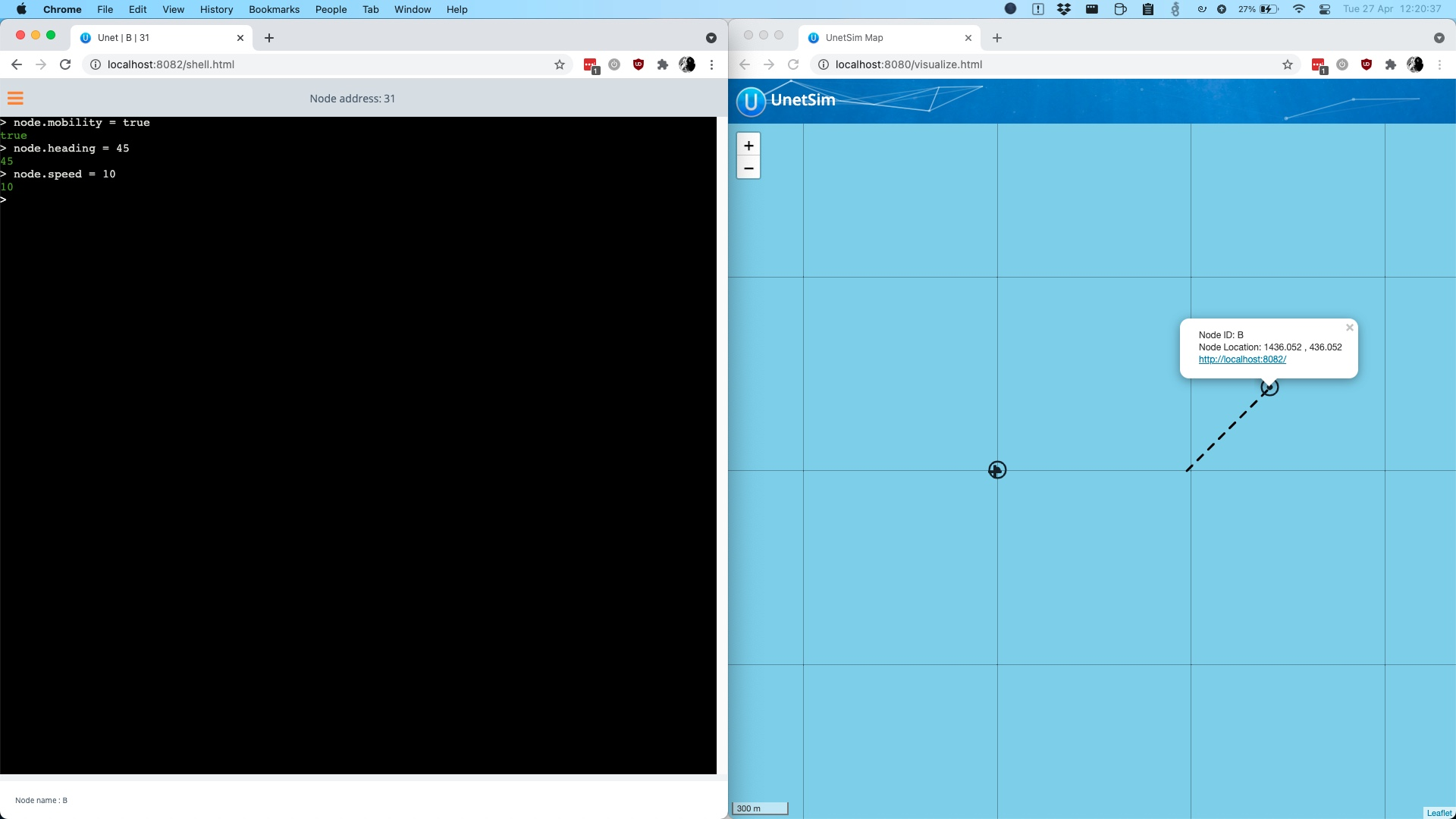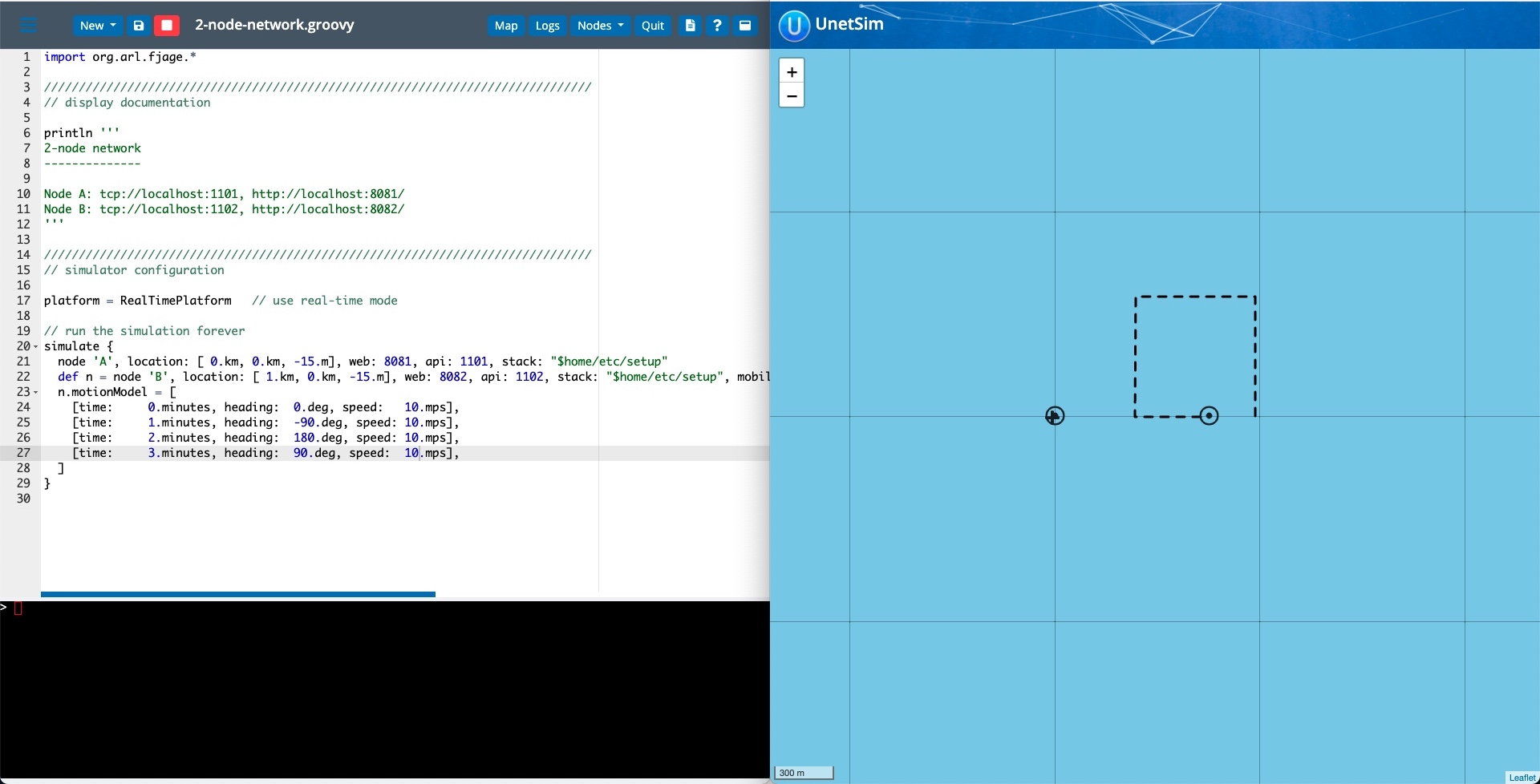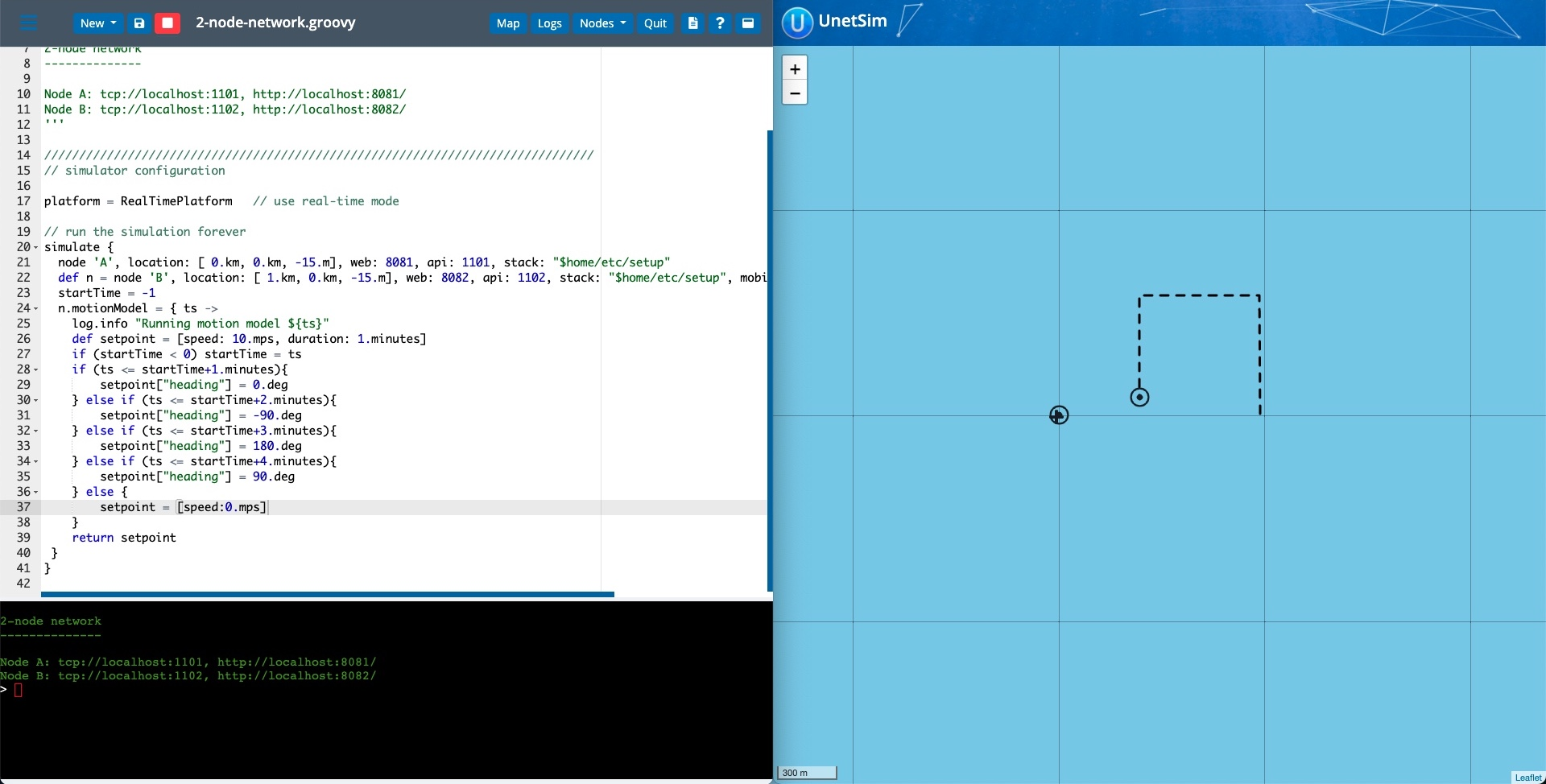The Unet simulator supports various ways of simulating the motion of the simulated nodes, from simple dynamics models to completely custom functions that can generate motion updates. Let’s look at how one can go about simulating the motion of nodes in the Unet Simulator.

## Dynamics Model

The NodeInfo agent (which is typically run in each simulated node) implements a basic dynamics model. The functionality can be turned on using the mobility parameter which can be set in a simulation script or directly on the NodeInfo agent in a running simulation.

When mobility is enabled, the NodeInfo agent automatically updates its location parameter based on motion parameters such as speed and heading using the simple dynamics model. This can be handy to simulate the motion of a node, for example, an AUV swimming away from an underwater modem.

Let’s try this out by manually updating the parameters of one of the nodes in a simulation, using the 2-node-network example from Unet IDE. The map view of the IDE can be used to visualize the motion of the nodes. Connect to the WebShell of Node B and set the mobility, heading, and speed parameters. As the simulation continues, the location of Node B will continue to be updated based on the speed and heading that you set.

For example, setting these values in the shell will cause the simulated node to as shown in the map view.

1
2
3
4
// On Node B's shell, set heading to 45deg and speed to 10m/s
node.mobility = true
node.speed = 10Most channel models in UnetStack take into account the distance between the nodes for calculating successful receptions. Hence simulating the motion of nodes in a network simulation can be very useful to measure and verify network behavior and performance more accurately.

## Setpoints

While the NodeInfo agent only exposes a basic dynamics model, the Unet simulation scripts allow for much more control by letting the user set a series of Setpoints of motion parameters on a node. This is done using the motionModel parameter on the node Object in the simulation script. The Unet Handbook has a few examples of this in Chapter 30.

Setting motionModel to a List of Maps with various motion properties for each of the Setpoint will automatically set the properties onto the NodeInfo agent in the simulated node at/for the appropriate time/duration. This in turn drives the dynamics model in the NodeInfo agent, giving the interpolated location between the Setpoints.

For example, a node moving in a square can be simulated using this simulation script using 4 Setpoints in the 2-node-network example. Note that the motion automatically stops after the last Setpoint.

1
2
3
4
5
6
7
def n = node 'B', location: [ 1.km, 0.km, -15.m], web: 8082, api: 1102, stack: "$home/etc/setup", mobility: true n.motionModel = [ [time: 0.minutes, heading: 0.deg, speed: 1.mps], [time: 1.minutes, heading: -90.deg, speed: 1.mps], [time: 2.minutes, heading: 180.deg, speed: 1.mps], [time: 3.minutes, heading: 90.deg, speed: 1.mps], ]The utility method MotionModel.lawnmower helps to generate this List for a set of Setpoint Map of properties for a lawnmower pattern of motion. ## Custom motion model Finally, if even more fine-grained motion control is required, one can set the property motionModel of the simulated node to a Groovy Closure. The closure is called by the simulator with the current simulation timestamp and is expected to return a single Setpoint in form of a Groovy Map. For example, a similar logic to a square pattern can be implemented with this closure assigned to motionModel in the 2-node-network example. 1 2 3 4 5 6 7 8 9 10 11 12 13 14 15 16 17 18 def n = node 'B', location: [ 1.km, 0.km, -15.m], web: 8082, api: 1102, stack: "$home/etc/setup", mobility: true
startTime = -1
n.motionModel = { ts ->
def setpoint = [speed: 10.mps, duration: 1.minutes]
if (startTime < 0) startTime = ts
if (ts <= startTime+1.minutes){
} else if (ts <= startTime+2.minutes){
} else if (ts <= startTime+3.minutes){
} else if (ts <= startTime+4.minutes){
} else {
setpoint = [speed:0.mps]
}
return setpoint
}


NOTE: Once a Setpoint is returned with its duration set to null, then it is assumed that the motion is complete and the simulator will stop calling the motionModel closure.More complex logic can also be implemented inside such closures including communicating with other Agents and even other nodes.

Finally, the closure based approach can also allow one to override the builtin dynamics model in the NodeInfo agent by simply returning a Setpoint with a location property which will allow the location of the node to be updated directly from the closure instead of going through the dynamics model. In such a mode, however, since there is no interpolation of motion parameters, the duration of each Setpoint would have to be small.

For example, we can implement a similar pattern as our first example by updating the location of the node every second.

1
2
3
4
5
6
7
8
9
def n = node 'B', location: [ 1.km, 0.km, -15.m], web: 8082, api: 1102, stack: "\$home/etc/setup", mobility: true
n.motionModel = { ts ->
def l = agentForService(org.arl.unet.Services.NODE_INFO).location
if (l != null){
l += 10*Math.sqrt(2)
l += 10*Math.sqrt(2)
}
return [duration: 1.seconds, location: l]
}